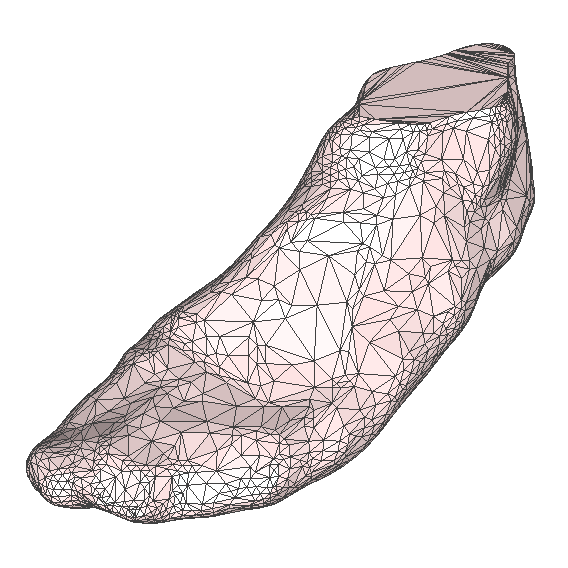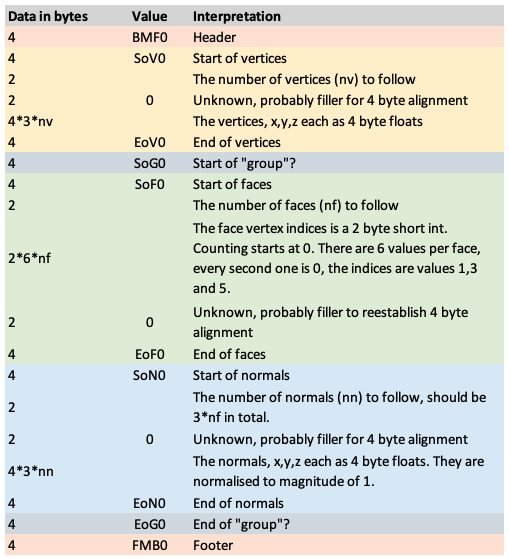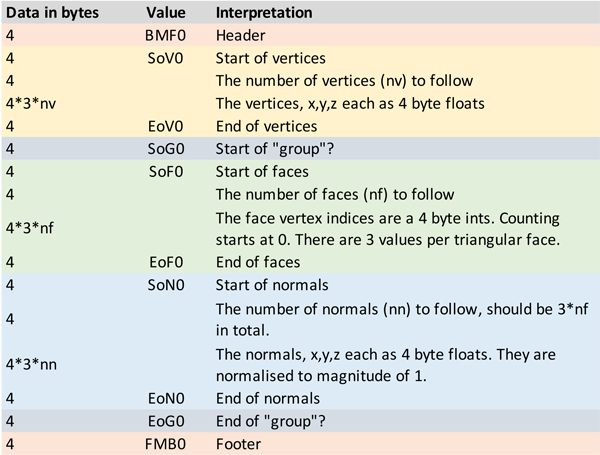# FleetFeet / Volumental BMF file format

Written by Paul Bourke
Original: December 2020. Updated: July 2022

The following defines the BMF format as used to represent foot scans by either, or both, FleetFeet and Volumental. The format specification is, as far as the author is aware, not made available publicly and what follows is a determination of the format using standard investigative methods. With the information presented here it is possible to use the feet models for purposes other than the limited capabilities of the companies products. As with all such investigations the format may be able to deal with other situations than are described here, but the information provided here deals with all the files currently examined.The files are required to define a 3D mesh which typically includes a list of the vertices and triangular faces. The faces are defined, as in other efficient formats, in reference to the vertex indices. This has the benefit of supporting shared vertices, similar to the OBJ format but unlike the STL format. This is exactly what the BMF format does, and it additionally defines a normal at each face vertex. The high level layout of the file is as follows:

• The number of vertices followed by a list of vertices
• The number of faces followed by a list of faces
• The number of normals followed by a list of the normals
• Footer

While not explicitly defined in the format, the units for the vertices are meters. More detail of the file format is shown in the figure below.Notes

• The x,y,z components of the vertices and normals are 4 byte floating point values.

• The face vertex indices are 2 byte short ints, mostly likely unsigned.

• Each data group (vertices, faces, normal) is surrounded by a start and end identifier. For example, SoV = Start of Vertices, SoF = Start of Faces, SoN = Start of Normals.

• The exact purpose of the SoG which is imagined to be Start of Group is unknown. It possibly allows for the identification of a different groups of faces within a single file. This is only a theory until a file is produced that has multiple face collections within the same file.

• The various instances of 2 byte short int values that are always 0 would only seem to serve to realign the file structure to 4 byte boundaries.

• The vertex indices defining the faces should only require 2 byte short ints but instead use a pair of 2 byte short ints. In all the files tested so far this second superfluous short int is always zero so it is also possible just a filler to realign to 4 byte boundaries.

• More usual for these types of 3D mesh descriptions would be to define a normal for each vertex, rather than for each vertex of a face. That is, shared vertices of a face would normally share the same normal. So the method used here is somewhat inefficient. It is postulated that there will always be 3 times the number of normals as faces, since each face is a triangle.

Sample file decomposed into sections, is shown below.

```Magic number header: "BMF0"
22064 ("V" "0" = 86 48)
21359 ("S" "o" = 83 111) Start of vertices
2550
0

22064 ("V" "0" = 86 48)
17775 ("E" "o" = 69 111) End of vertices
18224 ("G" "0" = 71 48)
21359 ("S" "o" = 83 111) Start of group
17968 ("F" "0" = 70 48)
21359 ("S" "o" = 83 111) Start of faces
5096

0
17968 ("F" "0" = 70 48)
17775 ("E" "o" = 69 111) End of faces?
20016 ("N" "0" = 78 48)
21359 ("S" "o" = 83 111) Start of Normals
15288
0

20016 ("N" "0" = 78 48)
17775 ("E" "o" = 69 111) End of Normals
18224 ("G" "0" = 71 48)
17775 ("E" "o" = 69 111) End of group
Magic number EOF: "FMB0"
```

Addendum - Based on further insights by Maarten de Vries

It seems the above discussion can be simplified if one realises that all integers are most likely 4 bytes instead of 2 bytes previously imagined. This makes sense since it eliminates the need for padding in the description above. That is, the number of vertices, number of faces, number of normals, and the vertex indices for the faces. Simplified and updated file structure shown below.Further notes

• All values are stored as little endian, so the 2 byte ints with 2 byte padding with 0 identified earlier are just 4 byte integers with values less that 65536.

• It is assumed all the integer values are 4 byte unsigned ints, probably academic since any single foot model is unlikely to ever contain more than 231 vertices or faces.